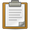This is a documentation subpage, where instructions, categories, or other information are stored for technical reasons. To view the Template page itself, see Template:US Census population.

This template is used as an information box on pages, showing each census year with a population, and a percent gain/loss comparison. See Alabama as an example of how this template is rendered.

### Usage

```{{US Census population
| 1790=
| 1800=
| 1810=
| 1820=
| 1830=
| 1840=
| 1850=
| 1860=
| 1870=
| 1880=
| 1890=
| 1900=
| 1910=
| 1920=
| 1930=
| 1940=
| 1950=
| 1960=
| 1970=
| 1980=
| 1990=
| 2000=
| estimate=
| estyear=
| estref=
| footnote=
}}```

### Alignment

The default alignment is to float on the right side of the page. To float it to the left, use `|align=left`. For center alignment, use `|align=center`. For left alignment, without wrapping text around it, use `|align=none`.